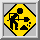# S114 FORMAT OF ASCII-FILESStill in progress !

Every Row contains the complete charge information of one event. The format of the files is :

```ENUM,Z1,Z2,Z3,Z4,Z5,Z6,Z7,Z8,Z9,Z10,Z11,Z12,ZB,ZB3
FORMAT (I8,14I4)

ENUM      = eventnumber
Z1 .. Z12 = charge of fragments in descending order (Z[i].ge.Z[i+1])
ZB        = Z_bound = sum_(Z[i].ge.2) Z[i]
ZB3       = sum_(Z[i].ge.3) Z[i]
```
For events with more than 12 detected fragments, the number and charges of these additional fragments can be calculated from the values of ZB and ZB3 (example in FORTRAN). If ZB_12 and ZB3_12 are the values calculated with only the first 12 fragments, the number of additional Z=2 particles NAL is

NAL = (ZB - ZB_12 - (ZB3-ZB3_12)).

For (ZB3-ZB3_12) = 3 one Z=3, = 4 one Z=4, = 5 one Z=5, = 6 two Z=3, = 7 one Z=4 and one Z=3, = 8 two Z=4, = 9 three Z=3 are left.

It is checked, that these reconstruction gives the correct set of charges for these events and that other possibilities, like (ZB3-ZB3_12)=9 -> one Z=9 or one Z=5 and one Z=4, do not occur in our samples.

Last updated: December 11th, 1995
Andreas Wörner

 Imprint ---- Data privacy protection ---- Haftungsausschluss